# 无限元在Abaqus静力分析中的应用-01-

1.1

Abaqus中用于静力分析的无限元是参考Zienkiewicz等人的工作建立的，此类无限元也被称为映射无限元，其特点为采用了两种形函数，即无限元的几何描述通过一组映射函数来实现，而位移场则与常规有限元相同，即采用标准的形函数来确定。

1.1.1 坐标的映射变换1 映射到自然坐标系的无限元

“极点（pole）”O相对于全局坐标的位置x0是任意的，一旦确定下来，则节点K的位置可表示为：2 形函数随自然坐标的变化

1.1.2 单元的位移模式

ξr的关系代入上式可得：

ξ趋近于1，即当r趋近于无穷远时，则位移u趋近于u3，如果令无穷远处的位移为零，即u3=0，则该无限元的形函数可重新表示为：

1.2

1.2.1 坐标的映射变换3 映射到自然坐标系的二维无限元

1 不同节点处各个映射函数的取值1.2.2 单元的位移模式

1.2.3 单元应力和应变

1.2.4 单元刚度矩阵

-02-

Abaqus中的无限元

2.1

Abaqus针对平面应力/应变、三维应力以及轴对称问题均提供了相应的无限元，对于Abaqus/Standard还可以使用带有减缩积分的无限元。此外Abaqus还提供有声学无限元。无限元在Abaqus单元库中遵循如图4所示的命名惯例。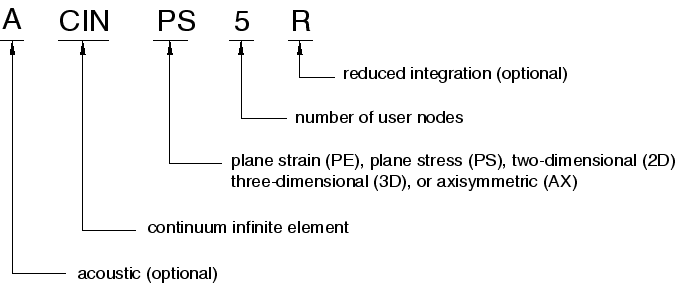4 Abaqus无限元命名惯例

2.2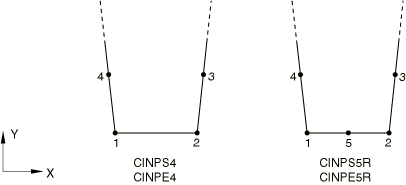a）平面应力/应变无限元b）轴对称无限元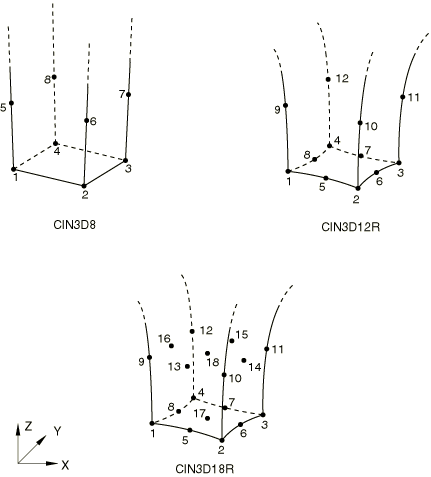c）三维无限元

5 Abaqus提供的无限元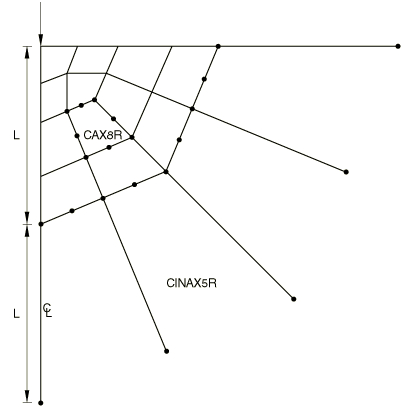6 作用在弹性半空间域中的点载荷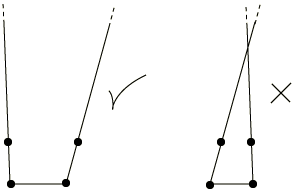7 可接受和不可接受的二维无限元

2.38 4节点平面单元节点定义

1, 6, 8, 1, 3

1, 1, 3, 6, 8

B点位于A点的逆时针方向，因此起始节点编号应为A点的节点编号，否则应为B点的节点编号。基于上述方法，本文通过MATLAB编制了无限元节点处理程序，可以基于待生成无限元的常规单元的单元号、近场边界节点号以及节点定义信息自动生成无限元的定义信息，程序及相应的建模过程参见附录。

2.49 含中心穿透裂纹的无限大板有限元模型

2.4.1 无限元布置方式对裂纹张开位移的影响a）布置方式1b）布置方式2

10 二维无限元布置方式

11给出了通过两种无限元布置方式获得的裂纹张开位移，从图中可以看到，两种布置方式得到的裂纹张开位移曲线差异较大，并且与解析解存在一定偏差，这说明布置方式1虽然易于实现，但并不能很好地模拟无限域边界。虽然布置方式2也与解析解存在较大偏差，但本文认为这主要是由于基体（常规单元划分的区域）尺寸设置较小所带来的精度损失。11 无限元布置方式对裂纹张开位移的影响

2.4.2 基体尺寸及极点位置对裂纹张开位移的影响12 基体尺寸及极点位置对裂纹张开位移的影响

12中可以看到，在小尺寸基体中，极点位置对计算结果存在很大的影响；而在大尺寸基体中，两种极点位置下的裂纹张开位移曲线完全吻合，并且与解析解非常接近，这说明计算结果对极点位置并不敏感。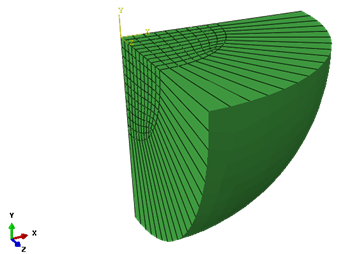13 含三维无限元的网格模型

 O. C. Zienkiewicz, C. Emson, P. Bettess. A novel boundary infinite element[J]. International journal for numerical methods in engineering, 1983, 19, 393-404.

 赵宇. 映射无限元在工程中的应用[D]. 广西: 广西大学, 1999.

 曾攀. 有限元分析及应用[M]. 北京: 清华大学出版社, 2004.

 Dassault Systemes. ABAQUS 6.14 Analysis user’s guide[M].

END

``clear,clc``%程序用于将Abaqus常规4节点平面单元转换为4节点单向映射无限元``%************************读取基本数据**************************************``%读取模型节点编号``Node_id_data=importdata("Node_id.txt");``%读取用于生成无限元的4节点平面单元的编号``Inf_elem_data=importdata("Inf_elem_node.txt");``%读取无限元近场边界(与常规单元相连的边界)上的节点(需要按顺序排列)``BC_node=importdata("BC_node.txt");``%整理边界节点数据为一维数组并剔除NaN数据``BC_node_num=size(BC_node,1)*size(BC_node,2)-sum(sum(isnan(BC_node)));``BC_node_data=zeros(BC_node_num,1);``k=1;``for i=1:size(BC_node,1)``  for j=1:size(BC_node,2)``    if isnan(BC_node(i,j))``      continue;``    else``      BC_node_data(k)=BC_node(i,j);``      k=k+1;``    end``  end``end``clear BC_node``%**************************************************************************``%*******************遍历4节点平面单元生成4节点无限元***********************``fileID=fopen("Inf_elem_def.txt","w");``Inf_elem_num=size(Inf_elem_data,1);``for i=1:Inf_elem_num``  Inf_elem_id=Inf_elem_data(i,1);``  %遍历当前单元的每一个节点 寻找属于近场边界的节点``  BC_node_current=zeros(2,1);``  k=1;``  for j=1:4``    if find(Inf_elem_data(i,j+1)==BC_node_data(:))``      BC_node_current(k,1)=Inf_elem_data(i,j+1);``      k=k+1;``    end``  end``  %根据逆时针编号顺序确定边界起始节点号``  %获取当前单元四个节点的坐标``  Node_Coord_X=zeros(4,1);``  Node_Coord_Y=zeros(4,1);``  for j=1:4``    %计算当前节点在节点定义信息中的索引号``    index=find(Inf_elem_data(i,j+1)==Node_id_data(:,1));``    Node_Coord_X(j)=Node_id_data(index,2);``    Node_Coord_Y(j)=Node_id_data(index,3);``  end``  %计算当前单元的中心坐标``  Node_Center_X=sum(Node_Coord_X)/4;``  Node_Center_Y=sum(Node_Coord_Y)/4;``  %计算当前单元位于边界的节点的坐标``  BC_node_Coord_X=zeros(2,1);``  BC_node_Coord_Y=zeros(2,1);``  for j=1:2``    index=find(BC_node_current(j)==Node_id_data(:,1));``    BC_node_Coord_X(j)=Node_id_data(index,2);``    BC_node_Coord_Y(j)=Node_id_data(index,3);``  end``  %计算边界节点与中心坐标形成的矢量``  BC_node_vector_I=[BC_node_Coord_X(1)-Node_Center_X;...``    BC_node_Coord_Y(1)-Node_Center_Y];``  BC_node_vector_J=[BC_node_Coord_X(2)-Node_Center_X;...``    BC_node_Coord_Y(2)-Node_Center_Y];``  %计算两个矢量的叉乘``  Cross_mult=(BC_node_vector_I(1)*BC_node_vector_J(2)-...``    BC_node_vector_I(2)*BC_node_vector_J(1));``  %根据叉乘正负判断无限元节点顺序编号的起始节点``  if Cross_mult>0``    BC_node_start=BC_node_current(1);``  else``    BC_node_start=BC_node_current(2);``  end``  %根据起始节点重新依照逆时针顺序进行编号``  Inf_elem_def=zeros(5,1);``  index_start=find(BC_node_start==Inf_elem_data(i,:));``  Inf_elem_def(1)=Inf_elem_data(i,1);``  Inf_elem_def(2:2+5-index_start)=Inf_elem_data(i,index_start:end);``  Inf_elem_def(8-index_start:end)=Inf_elem_data(i,2:index_start-1);``  %输出无限元定义信息``  for j=1:5``    if j==5``      fprintf(fileID,"%10d\n",Inf_elem_def(j));``    else``      fprintf(fileID,"%10d,",Inf_elem_def(j));``    end``  end``end``fclose(fileID);``%**************************************************************************``

来源：FEM and FEA
著作权归作者所有，欢迎分享，未经许可，不得转载
首次发布时间：2023-05-30
最近编辑：4月前
获赞 22粉丝 25文章 59课程 0还没有评论